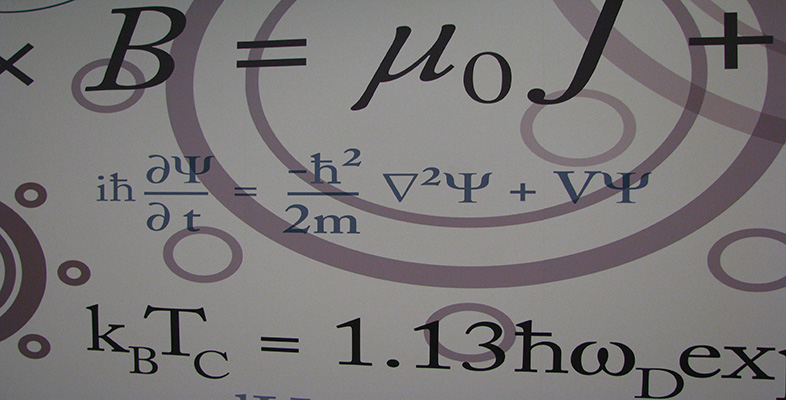Number systems

This free course is available to start right now. Review the full course description and key learning outcomes and create an account and enrol if you want a free statement of participation.

Free course

Number systems

Introduction

In this course we look at some different systems of numbers, and the rules for combining numbers in these systems. For each system we consider the question of which elements have additive and/or multiplicative inverses in the system. We look at solving certain equations in the system, such as linear, quadratic and other polynomial equations.

In Section 1 we start by revising the notation used for the rational numbers and the real numbers, and we list their arithmetical properties. You will meet other properties of these numbers in the analysis units, as the study of real functions depends on properties of the real numbers. We note that some quadratic equations with rational coefficients, such as x2 = 2, have solutions which are real but not rational.

In Section 2 we introduce the set of complex numbers. This system of numbers enables us to solve all polynomial equations, including those with no real solutions, such as x2 + 1 = 0. Just as real numbers correspond to points on the real line, so complex numbers correspond to points in a plane, known as the complex plane.

In Section 3 we look further at some properties of the integers, and introduce modular arithmetic. This will be useful in the group theory units, as some sets of numbers with the operation of modular addition or modular multiplication form groups.

In Section 4 we introduce the concept of a relation between elements of a set. This is a more general idea than that of a function, and leads us to a mathematical structure known as an equivalence relation. An equivalence relation on a set classifies elements of the set, separating them into disjoint subsets called equivalence classes.

This OpenLearn course is an adapted extract from the Open Unviersity course M208: Pure Mathematics [Tip: hold Ctrl and click a link to open it in a new tab. (Hide tip)]

M208_6